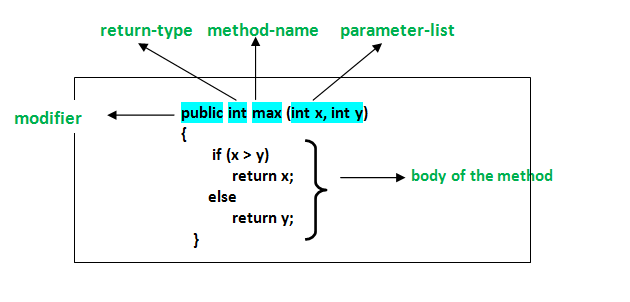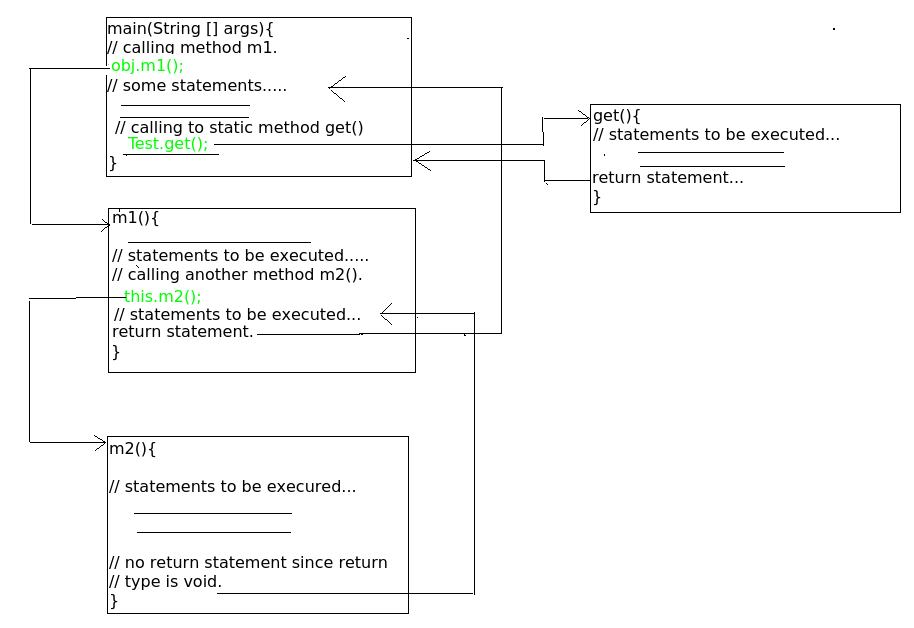Open in App
Not now

# Methods in Java

• Difficulty Level : Easy
• Last Updated : 21 Sep, 2022

Method in Java or Java Method is a collection of statements that perform some specific task and return the result to the caller. A Java method can perform some specific task without returning anything. Methods in Java allow us to reuse the code without retyping the code. In Java, every method must be part of some class that is different from languages like C, C++, and Python.

1. A method is like function i.e. used to expose behavior of an object.
2. it is a set of codes that perform a particular task.

Syntax: Declare a method

```<access_modifier> <return_type> <method_name>( list_of_parameters)
{
//body
}```

• Code Reusability
• Code Optimization

Note: Methods are time savers and help us to reuse the code without retyping the code.

### Method Declaration

In general, method declarations has six components :

1. Modifier: It defines the access type of the method i.e. from where it can be accessed in your application. In Java, there 4 types of access specifiers.

• public: It is accessible in all classes in your application.
• protected: It is accessible within the class in which it is defined and in its subclass/es
• private: It is accessible only within the class in which it is defined.
• default: It is declared/defined without using any modifier. It is accessible within the same class and package within which its class is defined.

2. The return type: The data type of the value returned by the method or void if does not return a value.

3. Method Name: the rules for field names apply to method names as well, but the convention is a little different.

4. Parameter list: Comma-separated list of the input parameters is defined, preceded with their data type, within the enclosed parenthesis. If there are no parameters, you must use empty parentheses ().

5. Exception list: The exceptions you expect by the method can throw, you can specify these exception(s).

6. Method body: it is enclosed between braces. The code you need to be executed to perform your intended operations.### Types of Methods in Java

There are two types of methods in Java:

1. Predefined Method: In Java, predefined methods are the method that is already defined in the Java class libraries is known as predefined methods. It is also known as the standard library method or built-in method. We can directly use these methods just by calling them in the program at any point.

2. User-defined Method: The method written by the user or programmer is known as a user-defined method. These methods are modified according to the requirement.

### Method Signature

It consists of the method name and a parameter list (number of parameters, type of the parameters, and order of the parameters). The return type and exceptions are not considered as part of it.

Method Signature of the above function:

` max(int x, int y) Number of parameters is 2, Type of parameter is int.`

### How to Name a Method?

A method name is typically a single word that should be a verb in lowercase or multi-word, that begins with a verb in lowercase followed by an adjective, noun….. After the first word, the first letter of each word should be capitalized.

Rules to Name a Method

• While defining a method, remember that the method name must be a verb and start with a lowercase letter.
• If the method name has more than two words, the first name must be a verb followed by an adjective or noun.
• In the multi-word method name, the first letter of each word must be in uppercase except the first word. For example, findSum, computeMax, setX and getX.

Generally, a method has a unique name within the class in which it is defined but sometimes a method might have the same name as other method names within the same class as method overloading is allowed in Java.

### Method Calling

The method needs to be called for using its functionality. There can be three situations when a method is called:
A method returns to the code that invoked it when:

• It completes all the statements in the method
• It reaches a return statement
• Throws an exception

Example:

## Java

 `// Java Program to Illustrate Methods`   `// Importing required classes` `import` `java.io.*;`   `// Class 1` `// Helper class` `class` `Addition {`   `    ``// Initially taking sum as 0` `    ``// as we have not started computation` `    ``int` `sum = ``0``;`   `    ``// Method` `    ``// To add two numbers` `    ``public` `int` `addTwoInt(``int` `a, ``int` `b)` `    ``{`   `        ``// Adding two integer value` `        ``sum = a + b;`   `        ``// Returning summation of two values` `        ``return` `sum;` `    ``}` `}`   `// Class 2` `// Helper class` `class` `GFG {`   `    ``// Main driver method` `    ``public` `static` `void` `main(String[] args)` `    ``{`   `        ``// Creating object of class 1 inside main() method` `        ``Addition add = ``new` `Addition();`   `        ``// Calling method of above class` `        ``// to add two integer` `        ``// using instance created` `        ``int` `s = add.addTwoInt(``1``, ``2``);`   `        ``// Printing the sum of two numbers` `        ``System.out.println(``"Sum of two integer values :"` `                           ``+ s);` `    ``}` `}`

Output

`Sum of two integer values :3`

Example 2:

## Java

 `// Java Program to Illustrate Method Calling` `// Via Different Ways of Calling a Method`   `// Importing required classes` `import` `java.io.*;`   `// Class 1` `// Helper class` `class` `Test {`   `    ``public` `static` `int` `i = ``0``;`   `    ``// Constructor of class` `    ``Test()` `    ``{`   `        ``// Counts the number of the objects of the class` `        ``i++;` `    ``}`   `    ``// Method 1` `    ``// To access static members of the class and` `    ``// and for getting total no of objects` `    ``// of the same class created so far` `    ``public` `static` `int` `get()` `    ``{`   `        ``// statements to be executed....` `        ``return` `i;` `    ``}`   `    ``// Method 2` `    ``// Instance method calling object directly` `    ``// that is created inside another class 'GFG'.`   `    ``// Can also be called by object directly created in the` `    ``// same class and from another method defined in the` `    ``// same class and return integer value as return type is` `    ``// int.` `    ``public` `int` `m1()` `    ``{`   `        ``// Display message only` `        ``System.out.println(` `            ``"Inside the method m1 by object of GFG class"``);`   `        ``// Calling m2() method within the same class.` `        ``this``.m2();`   `        ``// Statements to be executed if any` `        ``return` `1``;` `    ``}`   `    ``// Method 3` `    ``// Returns nothing` `    ``public` `void` `m2()` `    ``{`   `        ``// Print statement` `        ``System.out.println(` `            ``"In method m2 came from method m1"``);` `    ``}` `}`   `// Class 2` `// Main class` `class` `GFG {`   `    ``// Main driver method` `    ``public` `static` `void` `main(String[] args)` `    ``{`   `        ``// Creating object of above class inside the class` `        ``Test obj = ``new` `Test();`   `        ``// Calling method 2 inside main() method` `        ``int` `i = obj.m1();`   `        ``// Display message only` `        ``System.out.println(` `            ``"Control returned after method m1 :"` `+ i);`   `        ``// Call m2() method` `        ``// obj.m2();` `        ``int` `no_of_objects = Test.get();`   `        ``// Print statement` `        ``System.out.print(` `            ``"No of instances created till now : "``);`   `        ``System.out.println(no_of_objects);` `    ``}` `}`

Output

```Inside the method m1 by object of GFG class
In method m2 came from method m1
Control returned after method m1 :1
No of instances created till now : 1```

The control flow of the above program is as follows:Memory Allocation for Methods Calls

Methods calls are implemented through a stack. Whenever a method is called a stack frame is created within the stack area and after that, the arguments passed to and the local variables and value to be returned by this called method are stored in this stack frame and when execution of the called method is finished, the allocated stack frame would be deleted. There is a stack pointer register that tracks the top of the stack which is adjusted accordingly.

Related Articles: